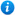# Adding and Subtracting Fractions - Year 5

KS2 Maths Teaching Resources: Adding and Subtracting Fractions - Year 5

42 slide PowerPoint presentation and 7 worksheets.

Year 5 maths programme of study - Number - fractions (including decimals)

• add and subtract fractions with the same denominators and denominators that are multiples of the same number

'Adding and Subtracting Fractions - Year 5' is a KS2 maths teaching resource ideal to use in a lesson covering the year 5 curriculum objective listed above. This colourful and engaging PowerPoint presentation can be used to introduce pupils to adding and subtracting fractions, or as a review of the topic. Content includes:

• Quick fraction recap including numerator and denominator explanations
• Explanation of how to add fractions with the same denominator
• Simplifying fractions and converting improper fractions to mixed numbers
• Adding fifths table activity and accompanying worksheet
• Adding fractions with the same denominator activity and worksheet
• Explanation of how to subtract fractions with the same denominator
• Subtracting fractions activity with accompanying worksheet
• Explanation of how to add and subtract fractions with different denominators
• Adding fractions with different denominators activity and worksheet
• Subtracting fractions with different denominators activity and worksheet
• Fraction word problems
• Link to a subtracting fractions interactive game
• Further worksheet

'Adding and Subtracting Fractions - Year 5' is fully editable so teachers can adapt the resource to suit their individual teaching requirements.

Preview 'Adding and Subtracting Fractions - Year 5' in full by clicking on the images above.

Our Price : £3.99 / 4 Credits## Get this resource as part of a bundle and save up to 73%## Year 5 Home Learning Pack - Maths and English

£19.99

By continuing to use the site, you agree to the use of cookies. You can change this and find out more by following this link